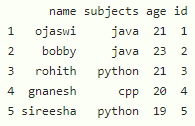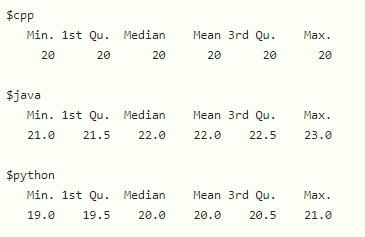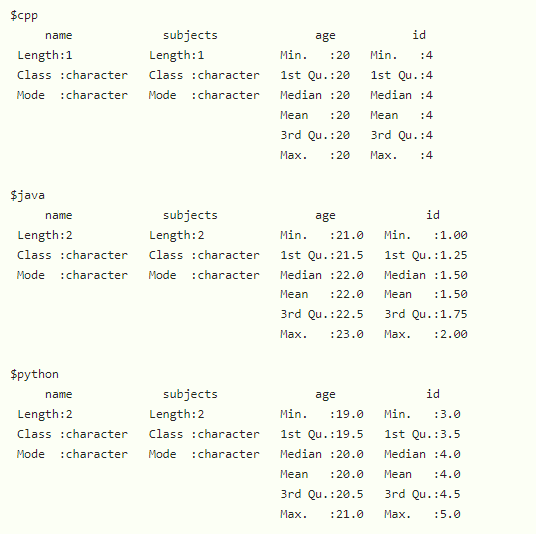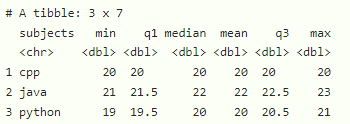GeeksforGeeks App
Open AppBrowser
Continue

# How to Calculate Summary Statistics by Group in R?

In this article, we will discuss how to calculate summary statistics by the group in the R programming language.

Summary statistics will return the following from the given data:

• Min – Minimum value in the given data
• 1st Quartile – first quartile in the data
• Median  – Median of the data
• Mean – Mean of the data
• 3rd Quartile – third quartile in the data
• Max – Maximum value in the given data

Let’s create the dataframe

## R

 `# create dataframe with 4 columns``data=``data.frame``(name=``c``(``"ojaswi"``,``"bobby"``,``"rohith"``,``"gnanesh"``,``"sireesha"``),``                ``subjects=``c``(``"java"``,``"java"``,``"python"``,``"cpp"``,``"python"``),``                ``age=``c``(21,23,21,20,19),``                ``id=``c``(1,2,3,4,5))` `# display``data`

Output:## Method 1: Using tapply() Function

In this method to calculate the summary statistics by group, the user needs to simply call the inbuilt tapply() function with the summary argument of this function passed with the given data for which the summary statistics is to be calculated, and under this method, user will take a summary function as the third parameter in the R language.

Syntax:

`tapply(data\$column_name, data\$group_column, summary) `

Parameters:

• data is the input dataframe
• column_name is the column to be summarized
• group_column is the column to be grouped
• summary is to get the summary data

Example:

In this example, we are going to display a summary by grouping subjects with age using the tapply() function with the summary argument in the R language..

## R

 `# create dataframe with 4 columns``data=``data.frame``(name=``c``(``"ojaswi"``,``"bobby"``,``"rohith"``,``"gnanesh"``,``"sireesha"``),``                ``subjects=``c``(``"java"``,``"java"``,``"python"``,``"cpp"``,``"python"``),``                ``age=``c``(21,23,21,20,19),``                ``id=``c``(1,2,3,4,5))` `# display summary by grouping subjects with age``tapply``(data\$age, data\$subjects, summary)`

Output:## Method 2: Using purrr Package:

In this method, the user has to first install and import the purr package, then the user has to follow the below syntax to calculate the summary statistics by a group of the given data in the R language.

Syntax to install and import the purr package in R console:

```install.package('purr')
library('purr')```

Syntax:

`data %>% split(.\$group_column) %>%map(summary) `

where,

• data is the input dataframe
• group_column is the column to be grouped
• summary is the function to get summary

Example:

Under this example, we are displaying a summary by grouping subjects with the help of the purr package in the R language.

## R

 `# load the library``library``(``"purrr"``)` `# create dataframe with 4 columns``data=``data.frame``(name=``c``(``"ojaswi"``,``"bobby"``,``"rohith"``,``"gnanesh"``,``"sireesha"``),``                ``subjects=``c``(``"java"``,``"java"``,``"python"``,``"cpp"``,``"python"``),``                ``age=``c``(21,23,21,20,19),``                ``id=``c``(1,2,3,4,5))` `# display summary by grouping subjects``data %>% ``split``(.\$subjects) %>%``map``(summary)`

Output:## Method 3: Using dplyr Package

In this approach, the user has to install and import the dplyr package in the working R console and then follow the below syntax with group_by and summarize() function to get summary by group in the R language.

Syntax to install and import the dplyr package in R console:

```install.package('dplyr')
library('dplyr')```

Syntax:

```data %>% group_by(group_column) %>% summarize(min = min(column),
q1 = quantile(column, 0.25),
median = median(column),
mean = mean(column),
q3 = quantile(column, 0.75),
max = max(column))```

Parameters:

• min(column) – to get the minimum of the column
• max(column) – to get the maximum of the column
• median(column) – to get the median of the column
• mean(column) – to get the mean of the column
• quantile(column, 0.25) – to get the first quartile of the column
• quantile(column, 0.75) – to get the third quartile of the column

Example:

In this example, we are displaying a summary by grouping subjects with age columns using the dplyr package in the R programming language.

## R

 `# load the library``library``(``"dplyr"``)` `# create dataframe with 4 columns``data=``data.frame``(name=``c``(``"ojaswi"``,``"bobby"``,``"rohith"``,``"gnanesh"``,``"sireesha"``),``                ``subjects=``c``(``"java"``,``"java"``,``"python"``,``"cpp"``,``"python"``),``                ``age=``c``(21,23,21,20,19),``                ``id=``c``(1,2,3,4,5))` `# display summary by grouping subjects with age column``data %>% ``group_by``(subjects) %>% ``summarize``(min = ``min``(age),``            ``q1 = ``quantile``(age, 0.25),``            ``median = ``median``(age),``            ``mean = ``mean``(age),``            ``q3 = ``quantile``(age, 0.75),``            ``max = ``max``(age))`

Output:My Personal Notes arrow_drop_up